## Tuesday, September 29, 2015

### Tetration Chaos

Here I've taken one of Sprott's simple three-dimensional chaotic flows with a quadratic nonlinearity (the system SQF, from table 3.1 reference ),
and replaced the quadratic nonlinearity with the tetration operator xx  to give the system:
The 2nd order tetration is realised on the analogue computer by multiplying the logarithm of |x| with |x| and then taking the exponential, this is all patched up as shown below:Initial conditions were (0,-0.5,0). The following pictures show the results from the analogue computer: pen plots on the left (600 seconds' worth), oscilloscope traces on the right (with computer in FAST mode):XZ-plane (left is pen plot (0.5 V/cm), right from oscilloscope (1 V/div))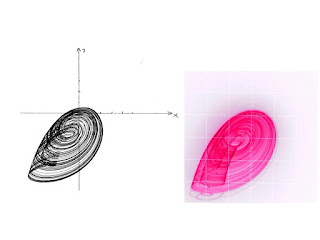XY-plane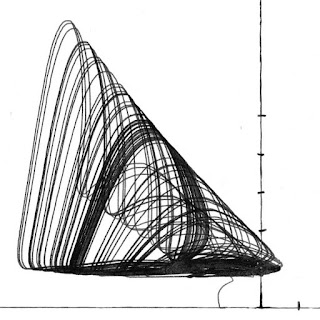YZ-plane (plotter)YZ-plane (oscilloscope)

And here are some MATHCAD results: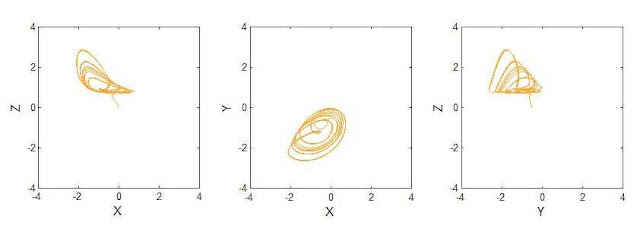MATHCAD results (∆t = 0.00025)

In passing, it's noted that the system has two equilibrium points, namely (-0.3463233..., -0.6926467..., 0.6926467...) and (-2, -4, 4), and the divergence of the system is equal to -1/2 and the system is dissipative.

It is apparent (and presumably not surprising) that many of the other chaotic systems remain chaotic when (for example) quadratic terms are replaced with |x||x|, e.g. Nosé–Hoover.

Reference

 J.C. Sprott, Elegant Chaos - Algebraically Simple Chaotic Flows, World Scientific, 2010, p. 70.

## Monday, July 20, 2015

### Hidden attractor / Invariant tori

The following interesting system is given by Sprott in :

Interesting because the system is either dissipative (exhibiting chaos) or conservative, dependent on the initial conditions.

Here's my patching for Sprott's system:

Results

The plotter results shown below were obtained with 0.2 µF integration capacitors throughout (with, as always, ×1 integrator inputs equating to 1 MΩ input resistances). The initial conditions were (2,0,0), corresponding to Sprott's first (red) solution .XY-plane (analogue computer left (570 seconds of trajectory), MATLAB right (100000 steps of 0.005 seconds))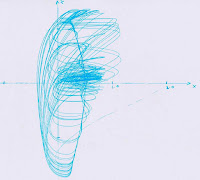XZ-plane (240 seconds of trajectory)YZ-plane (600 seconds of trajectory)

Interestingly, after a period of time (typically between 5 and 10 minutes ), the analogue computer result ceases being chaotic and settles into a stable(ish) periodic orbit - pretty much circular in the XY-plane, and corresponding (at least approximately) to the toroidal solution in Sprott's paper . This is more apparent when the computer is run in FAST mode, with the output to the oscilloscope. The following results were obtained with 2 second exposure times:Chaotic regime with periodic orbit. XY-plane. 0.5 V/div.Chaotic regime with periodic orbit. XZ-plane. 0.5 V/div.Chaotic regime with periodic orbit. YZ-plane. 0.5 V/div.

The trajectory starts in the (dissipative) chaotic regime (expected with the initial conditions assumed, according to ) but, after a period of time, collapses into the conservative regime, ending in a periodic (quasi?) orbit. The latter appears similar in location / size to the (outer dimension) of the invariant torus of , reached with initial conditions (1,0,0).

It's interesting that, the obvious circular orbit visible in the XY-plane is offset by an amount along the positive x-axis which is very close to 1/√2, which seems connected with the comment in  regarding the boundary between conservative and dissipative regions.

I am thinking that this eventual failure of the analogue computer's solution (and move from dissipative to conservative regime) may be either due to (a) offset nulls in various parts of the analogue computer effectively acting as sources of energy, or (b) due to losses in the integration capacitors.

Reference

 J. C. Sprott, 'A dynamical system with a strange attractor and invariant tori', Physics Letters A, 378, 2014, pp. 1361 - 1363.

## Monday, June 22, 2015

### The Aizawa attractor V

I now have an oscilloscope (a very nice HP 1740A). Here's the Aizawa attractor with computer in FAST mode (i.e. 1 nF integration capacitors) and a 2 second exposure:Aizawa attractor. 1 V/div both axes; vertical axis is z, horizontal is x. Note horizontal screen central axis is +1 V.Aizawa attractor. 1 V/div both axes; vertical axis is y, horizontal is x. Note horizontal screen central axis is 0 V.

## Thursday, June 18, 2015

### The Aizawa attractor IV

Not wishing to underestimate the power of the Dark Side, I've produced some renderings of the Aizawa attractor via MATLAB.

These were obtained using a very simple approach (Euler's method - the (x,y,z) coordinates of the current point are pushed through the differential equations, the resulting new values for the time-derivatives of (x,y,z) then multiplied by a time step, and then added to the current point, to give the next (x,y,z) point, and so on).

The following shows the xz-plane for a time step of 0.0001.

The published examples of the Aizawa attractor (for example, ) seem to use 0.01 as the time step - but it's apparent that the size of the time step has (perhaps not surprisingly) some effect on the solution.

The results below are for different time - step sizes (0.01, 0.001, 0.0001 seconds). In all cases the solutions show the first 600 seconds of the trajectory (i.e. for the 0.01 second time step, the equations were iterated 60,000 times, producing 60,000 points, and so on). The parameters are as in the previous posts (α = 0.95, β = 0.7, γ = 0.6, δ = 3.5, ε = 0.25 and ζ = 0.1), with initial conditions also as in previous post (x = 0.1, y = z = 0).

These tentative results tend to suggest that the 0.01 time step is a bit coarse: the 0.001 and 0.0001 cases look similar to each other, but different to the 0.01 case. Interestingly, the central tube-like structure becomes thicker (and more open) when the time step is smaller. Also shown is the attractor in the xy-plane.

The following shows the xy-plane for the 0.0001 case.

Evidently, the tube-like opening, although apparently circular, is not centred on the origin. This appears to agree with results obtained from the analog computer:xy-plane, 10 minutes' of trajectory

Reference

 http://jlswbs.blogspot.co.uk/2011/10/aizawa.html

## Tuesday, June 16, 2015

### The Aizawa attractor III

Here's my patching for the Aizawa attractor (see previous posts):

This uses all three multipliers, the divider (as a fourth multiplier), and the two squarers. The cubes are obtained directly using the (AD538 based) nth power circuits.

Amazingly, this all worked first time. Still sans oscilloscope - will be interested to see the attractor with the computer in FAST mode. In the meantime, here is the plotter output (1 µF integration capacitors). The plot shows the first ten minutes of the trajectory.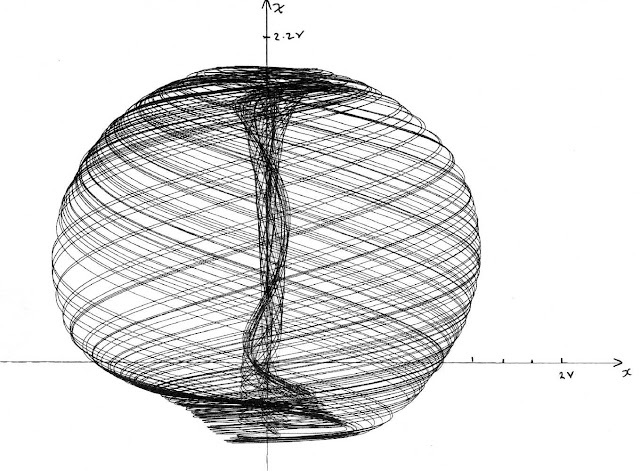2 V/cm both axes, 10 minutes' worth of trajectory.

Pleasingly, this agrees pretty well with other published images of the Aizawa attractor e.g. , although the latter appears to be an inverted mirror image of what I produced - perhaps the axes in  are reversed. The image of the attractor given in  does have the same handedness as mine.

Clearly, the attractor has some degree of symmetry about the z-axis. In the previous posts, I deduced that the attractor has three equilibrium points, lying along the z-axis:

Now, the first one (z = 1.9432644 V) does appear to correspond to the top of the attractor. But, the other two are well below the attractor. The bottom of the attractor is at about -0.58 V. This discrepancy requires some thought...

Reference

 http://www.algosome.com/articles/images/aizawa-attractor.jpg

 http://i.imgur.com/wMrmY.jpg

## Sunday, June 14, 2015

### The Aizawa attractor II

In the previous post, it was apparent (from numerical experiment) that the Aizawa attractor has three purely real equilibrium points along the z-axis, so long as parameter α is greater than a certain value, namely 0.932169751786... (with the other parameter values as listed in the previous post). If α is less than this value, then there is evidently one real equilibrium point, and two complex equilibrium points.

Here we derive an expression for α which gives the demarcation point between all-real and real and two complex equilibrium points.

The two (potentially) complex roots / equilibrium points are given by (see previous post):
Assuming that λ is not itself complex (see below), the above will roots will be purely real if λ2 = α (since this forces the right hand side of the above to be zero). From the previous post we have
Hence we need to solve
which, by inspection, has the solution

giving the value of 0.932169751786... which agrees with the value determined by numerical experiment.

Note that although the above gives the value for α for which the imaginary parts of the equilibrium points are exactly zero, it is not obvious that values of α greater than this will give purely real equilibrium points, since if α > α0 then λ will itself be complex, because 9γ2 - 4α3 will be negative, and the above argument / derivation of α0 assumes λ to be real.

### The Aizawa attractor I

There's a rather beautiful attractor called the Aizawa attractor: a sphere with a tube-like structure penetrating its axis  - a good candidate for the analog computer, in anticipation of the arrival of a new (old) oscilloscope from France.

The Aizawa attractor's equations are:

Given that there's precious little literature about this attractor, I have to plough my own furrow; I begin by searching for equilibrium points.

The game seems to be to set the left hand sides of the three equations to zero. Interestingly, by inspection, it's possible to solve the first two equations independently of the third, x = y = 0 being an obvious solution. In fact there are three solutions to the first pair of equations, obtainable, for example via:

Of these solutions, the first two can only yield real equilibrium points if x = y = 0; the third solution yields a complex equilibrium point, unless δ is zero or purely imaginary. If δ is zero then the first two equations would be decoupled. I am not sure what δ being imaginary would mean in terms of the analog computation - perhaps interpretable as a delay?

Given that equilibrium points should be real, since they exist in a real state space, we conclude that x = y = 0 for any such points - i.e. they must lie on the z-axis.

Setting x = y = 0 in the third equation, gives
which has three solutions:

It is not obvious that any of these roots are purely real. But, for certain values of  α and γ it transpires that they are...

...in all the published cases I can find, the Aizawa attractor uses the following parameter values: α = 0.95, β = 0.7, γ = 0.6, δ = 3.5, ε = 0.25 and ζ = 0.1, and plugging these values into the above equations gives the following (entirely real) roots for z:

Hence, for the parameter values listed, we expect three real equilibrium points, along the attractor's z-axis.

Now, clearly, λ depends solely on α and γ, and, as suspected, it's possible to adjust the value of (for example) α, so as to conjure up some complex values for z. A little experimentation shows that two of the roots become complex if α is reduced slightly, for example α = 0.93, yields:

which suggests just one (real) equilibrium point.

Indeed, it appears that the first root is always real, regardless of the value of α.

Further experimentation indicates that α  ≈ 0.932169751786 is the point at which the second and third roots start to have a non-zero imaginary part. This value is intriguingly close to the 0.95 value which seems to be the de facto value for α used for the Aizawa attractor. I assume this is not a coincidence, and that the attractor 'needs' the three (real) equilibrium points to exist. It would be interesting to know how the 0.95 value was found.

Vieta's formula for the sum of roots of a polynomial tells us that the sum of the three roots of
(i.e. equilibrium positions along the z-axis) must equal zero (since the quadratic term in z is zero). Hence, if one root (equilibrium position) is real, then the others must either both be real, or both be complex (with equal but opposite imaginary parts). It would appear not to be possible to have just a single complex root (which would mean exactly two real equilibrium positions).

Reference

 www.algosome.com/articles/aizawa-attractor-chaos.html